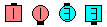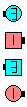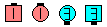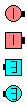Definitions of Square Dance Calls and Concepts

Index -->  Plus  |  A1  |  A2  |  C1  |  C2  |  C3A  |  C3B  |  C4  |  NOL  |
Definitions (Text Only) -->  Plus  |  A1  |  A2  |  C1  |  C2  |  C3A  |  C3B  |  C4  |  NOL  |
 Find call:

Wave \$B\$^\$?\$O(B Two-Faced Line \$B\$+\$i(B.

1 \$B\$D\$NF0\$-\$H\$7\$F(B, Lockit and Spread \$B\$r\$7\$^\$9(B.

Wave \$B\$^\$?\$O(B Two-Faced Line \$B\$G=*\$o\$j\$^\$9(B.

Wave \$B\$+\$i(B:Cross Lockit\$B\$NA0(B \$B8e(B
Two-Faced Line \$B\$+\$i(B:Cross Lockit\$B\$NA0(B \$B8e(B

\$B%@%s%9\$N%R%s%H(B: Centers \$B\$,(B Arm Turn 1/4 and Spread \$B\$r\$7(B, Ends \$B\$O(B slide in and Arm Turn 1/4 \$B\$r\$7\$^\$9(B. Ends \$B\$O(B Centers \$B\$,>l=j\$r6u\$1\$k\$^\$G(B, \$BF0\$-\$rBT\$D\$Y\$-\$G\$9(B.

Cross Lockit \$B\$O(B 2 \$B%Q!<%H\$N%3!<%k\$G\$O\$"\$j\$^\$;\$s\$N\$G(B, \$B%Q!<%H\$KJ,\$1\$F%3!<%k\$9\$Y\$-\$G\$O\$"\$j\$^\$;\$s(B. \$B\$3\$N%3!<%k\$O(B 1 \$B\$D\$N%9%`!<%9\$JF0\$-\$H\$7\$FMY\$i\$l\$^\$9(B. \$B\$3\$N%3!<%k\$N8e\$GA40w(B Roll \$B\$9\$k\$3\$H\$,\$G\$-\$^\$9(B.

Cross Lock The Hinge [C3B]: Cross Lockit, Hinge \$B\$G\$9!%(B

Hinge The Cross Lock [C3B]: Hinge, Cross Lockit \$B\$G\$9!%(B

anything The Cross Lock [C3B]: anything \$B\$N%3!<%k\$r\$7\$F(B; Cross Lockit \$B\$r\$7\$^\$9(B.Choreography for Cross LockitComments? Questions? Suggestions?

https://www.ceder.net/def/crosslockit.php?level=master&language=japan
26-October-2021 12:05:48